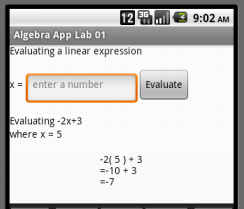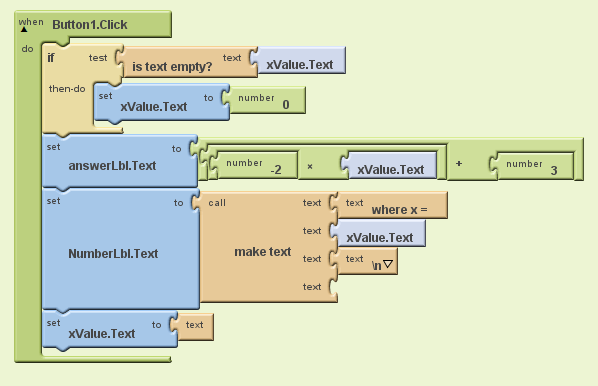## Algebra

Showing posts with label tutorial. Show all posts
Showing posts with label tutorial. Show all posts

### Free Algebra Study Guide with Videos

Welcome to OpenAlgebra.com, your comprehensive online destination for mastering algebra. Dive deep into our extensive collection of step-by-step tutorials, complemented by illustrative YouTube videos for a visual learning experience. Whether you're a student seeking supplemental material beyond your textbooks or a teacher looking for a rich resource of worked-out math problems, our platform offers a treasure trove of algebraic knowledge tailored to enhance your understanding.
 Chapter 1 - Real Numbers and Their Operations    1.1 Real Numbers and The Number Line    1.2 Adding and Subtracting Integers    1.3 Multiplying and Dividing Integers    1.4 Fractions    1.5 Review of Decimals and Percents    1.6 Exponents and Square Roots    1.7 Order of Operations    1.8 Sample Exam Questions Chapter 2 - Linear Equations and Inequalities    2.1 Introduction to Algebra    2.2 Simplifying Algebraic Expressions    2.3 Linear Equations: Part I    2.4 Linear Equations: Part II    2.5 Applications of Linear Equations    2.6 Ratio and Proportion Applications    2.7 Introduction to Inequalities and Interval Notation    2.8 Linear Inequalities (one variable)    2.9 Review Exercises and Sample Exam Chapter 3 - Graphing Lines    3.1 Rectangular Coordinate System    3.2 Graph by Plotting Points    3.3 Graph using Intercepts    3.4 Graph using the y-intercept and Slope    3.5 Finding Linear Equations    3.6 Parallel and Perpendicular Lines    3.7 Introduction to Functions    3.8 Linear Inequalities (Two Variables)    3.9 Review Exercises and Sample Exam Chapter 4 - Solving Linear Systems    4.1 Solving Linear Systems by Graphing    4.2 Solving Linear Systems by Substitution    4.3 Solving Linear Systems by Elimination    4.4 Applications of Linear Systems    4.5 Solving Systems of Linear Inequalities (Two Variables)    4.6 Review Exercises and Sample Exam    [ Elementary Algebra Exam #1 Solutions ]    [ Elementary Algebra Exam #2 Solutions ]    [ Elementary Algebra Exam #3 Solutions ]    [ Elementary Algebra Exam #4 Solutions ]     --- EA Sample Final Exam Ch. 1-7 ---Chapter 5 - Polynomials and Their Operations    5.1 Rules of Exponents (Integer Exponents)    5.2 Introduction to Polynomials and Evaluating    5.3 Adding and Subtracting Polynomials    5.4 Multiplying Polynomials and Special Products    5.5 Dividing Polynomials  (Synthetic Division)    5.6 Negative Exponents and Scientific Notation    5.7 Review Exercises and Sample Exam Chapter 6 - Factoring and Solving by Factoring    6.1 Introduction to Factoring    6.2 Factoring Trinomials    6.3 Factoring Trinomials ax^2 + bx + c    6.4 Factoring Binomials    6.5 General Guidelines for Factoring Polynomials    6.6 Solving Equations by Factoring    6.7 Applications involving Quadratic Equations    6.8 Review Exercises and Sample Exam Chapter 7 - Rational Expressions and Equations    7.1 Simplifying Rational Expressions    7.2 Multiplying and Dividing Rational Expressions    7.3 Adding and Subtracting Rational Expressions    7.4 Complex Fractions    7.5 Solving Rational Equations    7.6 Applications of Rational Equations    7.7 Variation    7.8 Review Exercises and Sample Exam Do you find this cool math site helpful? If so please share the link or embed in your mylab math course. Also, feel free to copy-and-paste anything you find useful here.  All we ask is that you link back to this site:  OpenAlgebra.com

Embark on an advanced journey with our Intermediate Algebra (IA) section, also known as Algebra 2. Delve into intricate topics, unravel complex equations, and enhance your mathematical prowess. Our meticulously crafted resources, combined with illustrative video tutorials, ensure a deeper understanding of algebraic concepts, bridging the gap between foundational knowledge and advanced applications. Whether you're gearing up for a challenging exam or simply eager to expand your algebraic horizons, our Intermediate Algebra section is tailored to meet your needs.